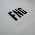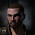## Wednesday, November 09, 2011

### Tracking versus Transversal, Signature Resolution versus Signature Radius

In my post last week commenter Suleiman Shouaa pointed out that I was pretty much ignoring the relationship between signature size and ability to hit:
So you're saying that a Megathron cannot track another Battleship MWDing at 15km? Wow, that's a pretty impressive amount of misinformation coming out of a single sentence....
Yes, mea culpa. I did completely ignore that MWD increases the size of the target's signature and this not only makes it easier to target quickly, it also helps negate the advantages that moving fast transversally has against tracking.

My error lies in that I think of the two separately but the formula to determine hits has them combined in the equation. From Evelopedia:

The way I was thinking is that the tracking versus transversal part merely determines if your guns can move fast enough to aim at the target; then assuming you can aim at the target, then the resolution versus radius to determine the dispersion of the shot versus the size of the target area profile.

But its not a two part process, its a one step calculation and turning on a MWD may increase transversal speed but it also increases the radius. So the question is, how much effect does the two changes have?

(Side Note: I use "transversal" velocity but the correct value to use in the calculations is "Angular" velocity which measures apparent movement of something in radians per second.)

So let's go back to our Megathron versus Maelstrom example, using current blaster values. Let's also assume that we are using Caldari Navy ammo in Neutron Blaster Cannon IIs, and that the Maelstrom is at optimal so the second part of the equation is zero. The stock Maelstrom (skills all level V) moves at a top speed of 118 m/s and a sig radius of 460 meters; with the MWD on it moves at 835 m/s and a radius of 2760 meters. The blaster on the Megathron has a tracking of 0.07442 radians/sec and a signature resolution of 400 meters.

So what's the angular velocity of the Maelstrom? Well, assuming the ship remains at optimal range, the a single radian is 4500 meters (i.e. optimal range of the blaster) and thus the angular velocity is 0.02622 rad/second at normal top speed and 0.6133 rad/second at MWD speed. EDIT See below.

So chance to hit Maelstrom without MWD on = 0.5 ^ (( (0.02622/0.07442) x (400/460) )^2 )
= 0.5 ^ ( (0.3523 x 0.8696) ^ 2)
= 0.5 ^ (0.3064 ^ 2)
= 0.5 ^ 0.0939
= 0.9370

And chance to hit once that Maelstrom punches the MWD button?
0.5 ^ ( ((0.6133/0.07442) x (400/2760)) ^ 2)
= 0.5 ^ ( (8.2411 x 0.1449) ^2)
= 0.5 ^ (1.1941^2)
= 0.5 ^ 1.4259
= 0.4529

So at optimal range, the chance to hit of the Megatron towards the Maelstrom with MWD on is halved. But Suleiman's complaint was that I was implying that at 15 km the Megathron could not hit the Maelstrom (and to be fair, that is what I intended to imply) so let's see what the math says.

EDIT:
As Luccul pointed out below, I got the angular velocity wrong with MWD on. Looks like I used the sig radius as the velocity, sigh. The actual angular velocity is 0.1856 to give us:

And chance to hit once that Maelstrom punches the MWD button?
0.5 ^ ( ((0.1856/0.07442) x (400/2760)) ^ 2)
= 0.5 ^ ( (2.4940x 0.1449) ^2)
= 0.5 ^ (0.3614^2)
= 0.5 ^0.1306
= 0.9135

So, as everyone keeps pointing out to me, the 500% increase in speed is cancelled out by the 500% increase in signature. Gah.
:END EDIT

At 15000 meters the angular velocity of the Maelstrom is 0.00787 r/s normally and 0.05567 r/s with MWD. We need to take the second part of the equation into play now so fall off range is 13000 meters.

No MWD at 15km:
0.5 ^ ((( (0.00787 / 0.07442) x (400/460) ) ^2 + ((15000 - 4500) / 13000 )^2)
= 0.5 ^ ( (0.1058 x 0.8696 )^2 + ( 0.8077)^2   )
= 0.5 ^ ( 0.00846 + 0.6524 )
= 0.6325

And MWD at 15 km:
0.5 ^ ((( ( 0.05567 / 0.07442) x (400/2760 ) ) ^2 + ((15000 - 4500) / 13000 )^2)
= 0.5 ^ ( (0.7481 x 0.1449 )^2 + ( 0.8077)^2   )
= 0.5 ^ ( 0.01175 + 0.6524 )
= 0.6311

So assuming my math is correct (and I'm running out of time to check my calculations so someone please comment if I'm wrong), the extra velocity at 15 km is negated by the ballooning size of the signature. In close ranges (i.e. optimal), the MWD speed makes a significant difference.

Now the question is, how much does that 20% extra tracking actually help blasters?

A big thanks to Suleiman Shouaa for pointing out my glaring mistake.

1.Maths :(

2.Anonymous7:04 pm

You're making it harder than it needs to be. A MWD will make you go roughly 5 times as fast, but balloon your signature to 500%. If you're orbiting, those increases cancel each other out. If you're approaching or escaping, you're left with the sig radius increase, and more wrecking shots.

3.Fuck math

4.Yay maths!

Looking at your optimal with MWD calculation, (0.5^1.4259)=0.372, not 0.4529. With a 20% increase, (assuming that means tracking equal to 0.089304) I'm getting 95% and 50%, which isn't much for the sans MWD, but a pretty sizable increase versus the MWDing target.

As serpentinelogic above me points out, the angular velocity that's being used is an absolutely perfect circle which will never be the case in game. So, in practice these hit percentages would represent a lower limit rather than an actual to-hit chance.

Oh, and a 20% change wouldn't affect anything at 15km...still 63%ish. They could up the tracking by a million and it would still be 63%. Hope that helps...and that my maths isn't wrong.

5.Yes, I realize its a very specific worst case scenario. The point was to demonstrate the relative effectsof the mwd in the two cases. I will double check the math tomorrow.

6.Anonymous12:20 am

Check your MWD angular velocity number for MWD at optimal. Angular = arc length / radius, so that's 835/4500 = 0.1856. Redoing the MWD calculation:
0.5 ^ ( ((0.1856/0.07442) x (400/2760)) ^ 2)
= 0.5 ^ ( (2.494 x 0.1449) ^ 2)
= 0.5 ^ 0.1306
= 0.913, which is not much different than the non-MWD calculation.

But that stands to reason - as serpentinelogic pointed out, 5 times the speed cancels 5 times the sig in the equation.

7.Ok, fixed my terribad math in the post. Suffice to say, everyone else is right.

8.You would not need all that calculating. If you have the formula, it doesnt matter what all the values were. If all values except for angular velocity and target signature stay the same and the target signature increase by a factor of 5, then the angular velocity needs to be increased by 5 as well to cancel each other. Sadly, you will virtually never be able to turn the 500% topspeed in 500% angular velocity as only very few ships can orbit at their full mwd speed.
That means, that in no ship and against no turret (same is true for missiles) you can evade damage with a mwd.
It can help to dictate range and get away and whatever, but it never helps to not get hit.

9.So, an MWD on a PvP-boat is useless for speed-tanking? Did I get that right?

10.Correct. Its good for range control but the speedbonus does not help otherwise.

11.Oh wow... and i learn that NOW, after all these years... :D Thanks for enlightening me Kirith ;D

12.That's right, I am completely satisfied with you that math involve in every stream of learning and in life style too, like money counting, share market and daily shopping.I am here to discuss a simple definition regarding arc length as-Arc is a curve. Length or an arc is known as arc length. .Arc length is described as measurement of distance along the curved.
Finding Arc Length